# RD Sharma Solutions for Class 10 Maths Chapter 11 Constructions Exercise 11.3

The main topic covered in this exercise is the Construction of tangents to a circle. By referring to RD Sharma Solutions Class 10 students can get to know the right steps in encountering tough problems with ease. These solutions are prepared according to the CBSE standard to match the needs of the student. For better clarity on how to answer questions of this exercise, the RD Sharma Solutions for Class 10 Maths Chapter 11 Constructions Exercise 11.3 PDF is available for students below.

## RD Sharma Solutions for Class 10 Maths Chapter 11 Constructions Exercise 11.3 Download PDF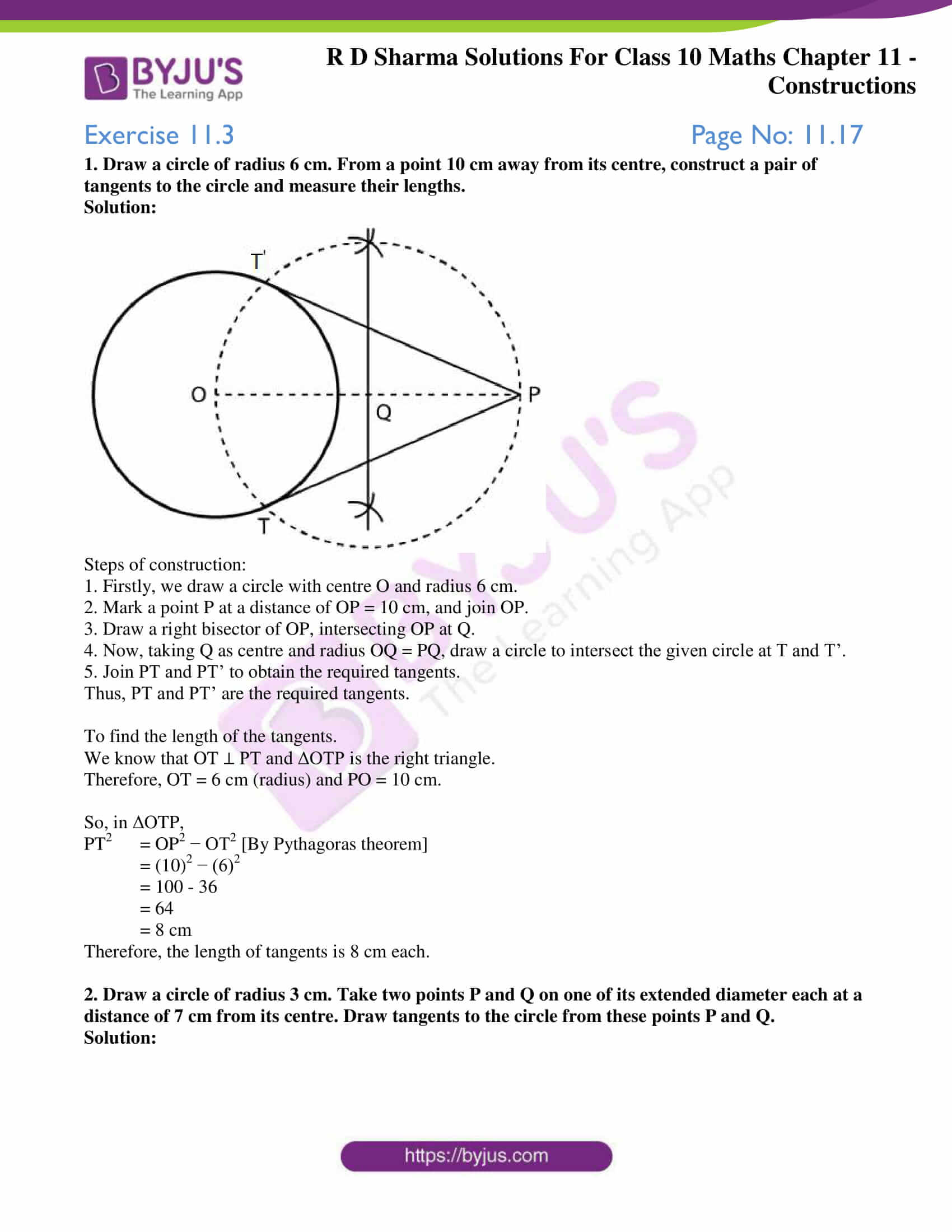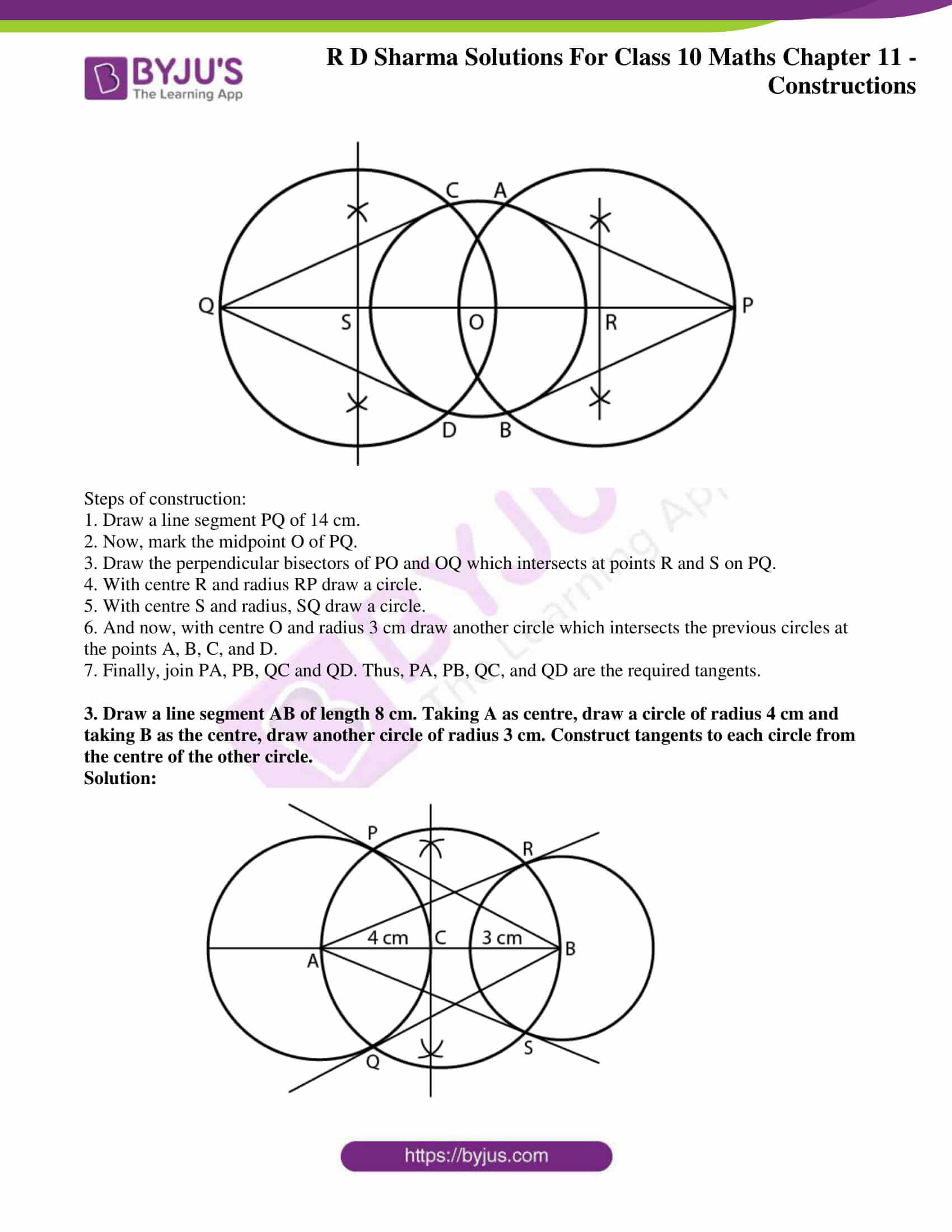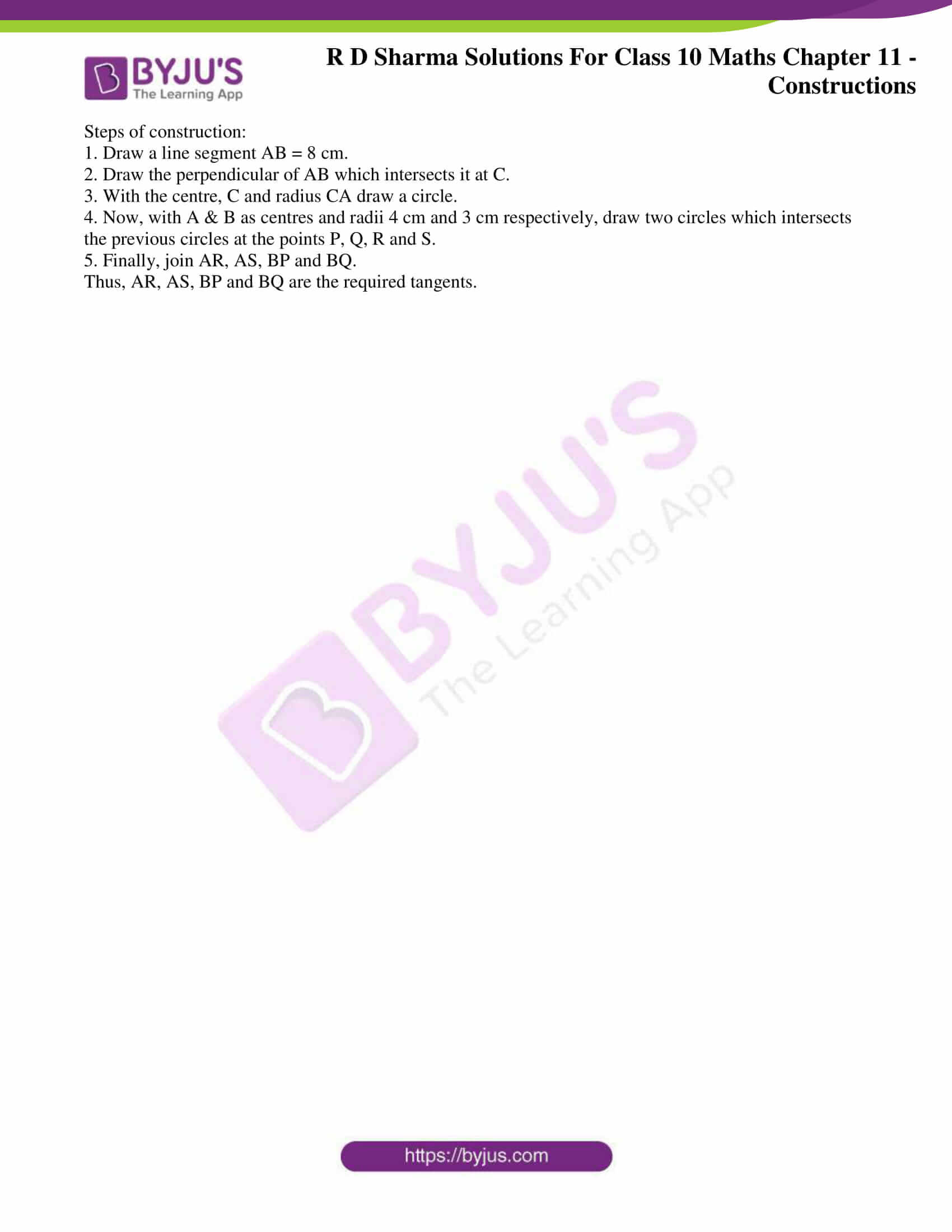### Access Answers to Maths RD Sharma Solutions for Class 10 Chapter 11 Constructions Exercise 11.3

1. Draw a circle of radius 6 cm. From a point 10 cm away from its centre, construct a pair of tangents to the circle and measure their lengths.

Solution: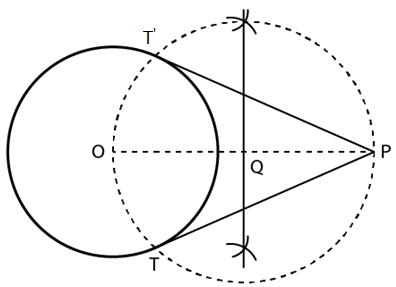Steps of construction:

1. Firstly, we draw a circle with centre O and radius 6 cm.

2. Mark a point P at a distance of OP = 10 cm, and join OP.

3. Draw a right bisector of OP, intersecting OP at Q.

4. Now, taking Q as centre and radius OQ = PQ, draw a circle to intersect the given circle at T and T’.

5. Join PT and PT’ to obtain the required tangents.

Thus, PT and PT’ are the required tangents.

To find the length of the tangents.

We know that OT ⊥ PT and ΔOTP is the right triangle.

Therefore, OT = 6 cm (radius) and PO = 10 cm.

So, in ΔOTP,

PT2  = OP2 − OT2 [By Pythagoras theorem]

= (10)2 − (6)2

= 100 – 36

= 64

= 8 cm

Therefore, the length of tangents is 8 cm each.

​2. Draw a circle of radius 3 cm. Take two points P and Q on one of its extended diameter each at a distance of 7 cm from its centre. Draw tangents to the circle from these points P and Q.

Solution: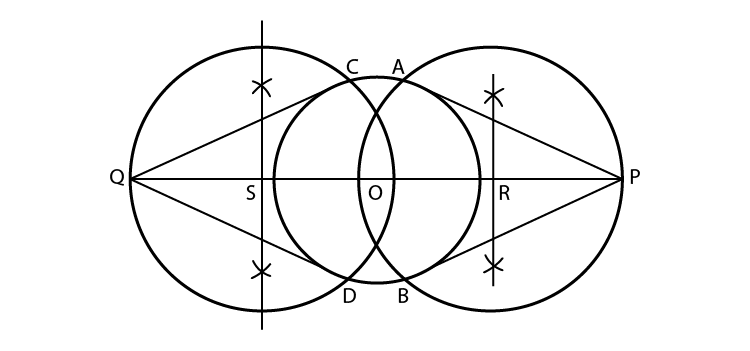Steps of construction:

1. Draw a line segment PQ of 14 cm.

2. Now, mark the midpoint O of PQ.

3. Draw the perpendicular bisectors of PO and OQ which intersects at points R and S on PQ.

4. With centre R and radius RP draw a circle.

5. With centre S and radius, SQ draw a circle.

6. And now, with centre O and radius 3 cm draw another circle which intersects the previous circles at the points A, B, C, and D.

7. Finally, join PA, PB, QC and QD. Thus, PA, PB, QC, and QD are the required tangents.

​3. Draw a line segment AB of length 8 cm. Taking A as centre, draw a circle of radius 4 cm and taking B as the centre, draw another circle of radius 3 cm. Construct tangents to each circle from the centre of the other circle.

Solution: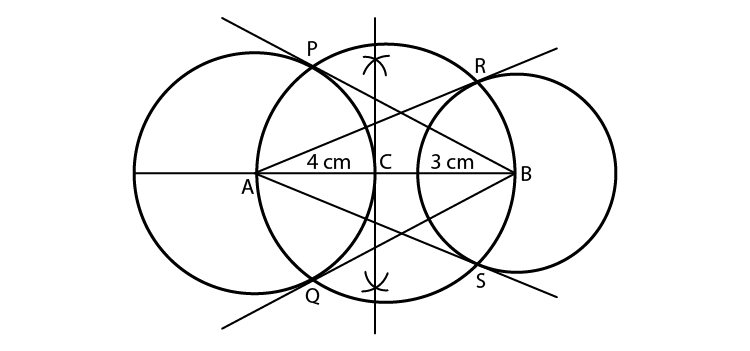Steps of construction:

1. Draw a line segment AB = 8 cm.

2. Draw the perpendicular of AB which intersects it at C.

3. With the centre, C and radius CA draw a circle.

4. Now, with A & B as centres and radii 4 cm and 3 cm, respectively, draw two circles which intersects the previous circles at the points P, Q, R and S.

5. Finally, join AR, AS, BP and BQ.

Thus, AR, AS, BP and BQ are the required tangents.